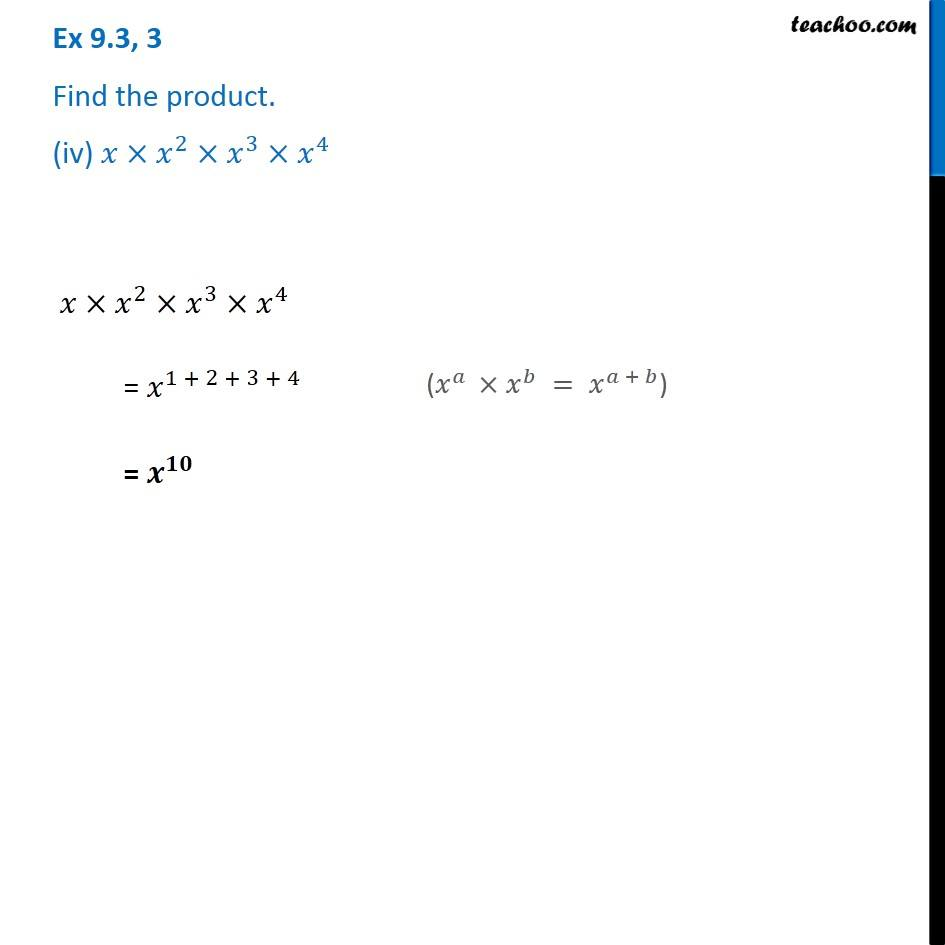1. Chapter 9 Class 8 Algebraic Expressions and Identities
2. Serial order wise
3. Ex 9.3

Transcript

Ex 9.3, 3 Find the product. (iv) 𝑥×𝑥^2×𝑥^3×𝑥^4 𝑥×𝑥^2×𝑥^3×𝑥^4 = 𝑥^(1 + 2 + 3 + 4) = 𝒙^𝟏𝟎 (𝑥^𝑎 ×𝑥^𝑏 = 𝑥^(𝑎 + 𝑏))